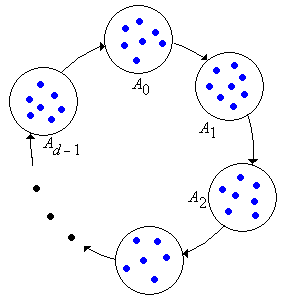]> Periodicity

## 3. Periodicity

A state in a Markov chain is periodic if the chain can return to the state only at multiples of some integer larger than 1. Periodic behavior complicates the study of the limiting behavior of the chain. As we will see in this section, we can eliminate the periodic behavior by considering the $d$-step chain, where $d$ is the period, but only at the expense of introducing additional equivalence classes. Thus, in a sense, we can trade one form of complexity for another.

### Basic Theory

As usual, our starting point is a (time homogeneous) Markov chain $X X 0 X 1 X 2$ with countable state space $S$ and transition probability matrix $P$. The period of state $x S$ is

$d x n P n x x 0$

Thus, starting in $x$, the chain can return to $x$ only at multiples of the period $d$, and $d$ is the largest such integer. State $x$ is aperiodic if $d x 1$ and periodic if $d x 1$. Perhaps the most important result is that period, like recurrence and transience, is a class property.

Show that if $x y$ then $d x d y$.

1. Suppose that $x y$. The result is trivial if $x y$, so let's assume that $x y$
2. Recall that there exist positive integers $j$ and $k$ such that $P j x y 0$ and $P k y x 0$. Show that $P j k x x 0$ and hence $d x j k$
3. Suppose that $n$ is a positive integer with $P n y y 0$. Show that $P j k n x x 0$ and hence $d x j k n$
4. From (b) and (c), conclude that $d x n$
5. Now conclude from the definition of period that $d y d x$
6. Reverse the roles of $x$ and $y$ to conclude that $d x d y$
7. Conclude that $d x d y$

Thus, the definitions of period, periodic, and aperiodic apply to equivalence classes as well as individual states. When the chain is irreducible, we can apply these terms to the entire chain.

Suppose that $x S$.

1. Show that if $P x x 0$ then $x$ (and hence the equivalence class of $x$) is aperiodic.
2. Sketch a state diagram to show that the converse of (a) is not true.

#### The Cyclic Classes

Suppose now that $X X 0 X 1 X 2$ is irreducible and is periodic with period $d$. There is no real loss in generality in assuming that the chain is irreducible, for if this were not the case, we could simply restrict our attention to one of the closed, irreducible equivalence classes. Now, we fix a reference state $u S$, and for $k 0 1 d 1$, define

$A k x S n P n d k u x 0$

Suppose that $j$ and $k$ are indices in $0 1 d 1$. Show that $x A j$ and $y A k$ if and only if $P n x y 0$ for some $n k j d$.

Show that $A 0 A 1 A d 1$ are the equivalence classes for the $d$-step to and from relation $d$ that governs the $d$-step chain $X 0 X d X 2 d$ that has transition matrix $P d$

In particular, $A 0 A 1 A d 1$ partitions the state space $S$; these sets are known as the cyclic classes. The basic structure of the chain is shown in the state diagram below:### Examples and Special Cases

Consider the Markov chain with state space $S 1 2 3 4 5 6 7$ and transition matrix $P$ given below:

$P 0 0 12 14 14 0 0 0 0 13 0 23 0 0 0 0 0 0 0 13 23 0 0 0 0 0 12 12 0 0 0 0 0 34 14 12 12 0 0 0 0 0 14 34 0 0 0 0 0$
1. Sketch the state digraph and show that the chain is irreducible.
2. Find the period $d$.
3. Find $P d$.
4. Identify the cyclic classes.

#### Special Models

Review the definition of the basic Ehrenfest chain. Show that this chain has period 2, and find the cyclic classes.

Review the definition of the modified Ehrenfest chain. Show that this chain is aperiodic.

Review the definition of the simple random walk on $k$ . Show that the chain is periodic with period 2, and find the cyclic classes.